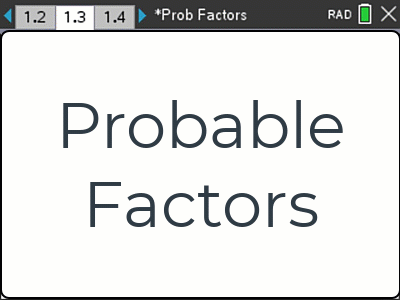# Activities

•• ##### AuthorAust Senior

2 Hours

• ##### Device
•TI-Nspire™ CX

5.0

## Probable Factors#### Activity Overview

What is the probability a randomly generated quadratic will factorise? This investigation looks at a substantially reduced set of equations. Dice are used to determine the coefficients. The investigation starts by using a simulation and reducing the set further by considering integer factors. The activity is a wonderful mix of algebra and probability, with extension options available for sampling distributions. A great option for a Problem Solving and Modelling Task.

#### Objectives

Students set up a simulation to help evaluate the reasonableness of pending theoretical calculations and solutions. Students draw from a mixture of mathematical concepts and procedures from previous units to help explore the problem. Students use a mixture of data, statistics, graphs, algebra and logic to support their findings. The nature of the task means that all student work will be unique!